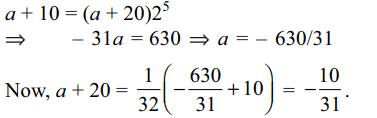## Sequence and Series Questions and Answers Part-7

1. Let $n\epsilon N$ , n > 25. Let A, G, H denote the arithmetic mean, geometric mean and harmonic mean of 25 and n. The least value of n for which A, G, $H\epsilon$ {25, 26, ...., n}, is
a) 49
b) 81
c) 169
d) 225

Explanation: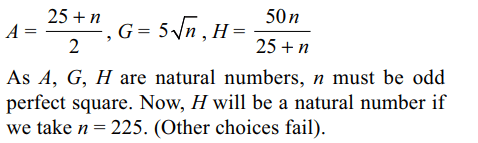2. Sum to n terms of the series $\frac{6}{5}+\frac{27}{25}+\frac{128}{125}+\frac{629}{625}+...$
is
a) $n+\frac{5}{16}\left(1-\frac{1}{5^{n}}\right)-\frac{n}{4\left(5^{n}\right)}$
b) $n+\frac{5}{16}\left(1-\frac{1}{5^{n}}\right)-\frac{1}{20}$
c) $n+\frac{5}{16}\left(1-\frac{1}{5^{n}}\right)-\frac{n}{20}$
d) none of these

Explanation: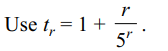3. Let a, b, c be three distinct positive numbers which are G.P. If $\log_{c}a,\log_{b}c,\log_{a}b$    are in A.P., then the common difference of the A.P. is
a) 1/2
b) 3/2
c) 5/2
d) 7/2

Explanation: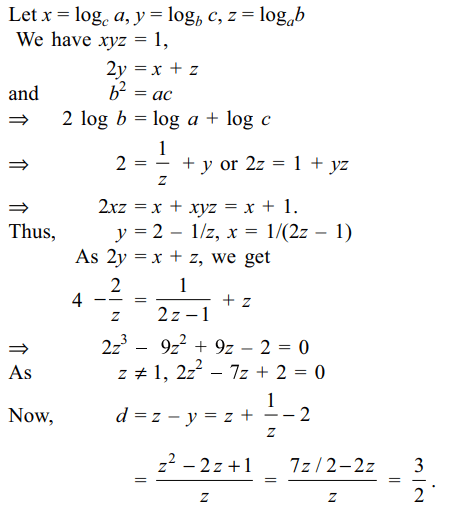4. If a, b, c are in G.P. then $\frac{b-a}{b-c}+\frac{b+a}{b+c}$    equals
a) 0
b) -1
c) ab
d) $a^{2}-b^{2}$

Explanation: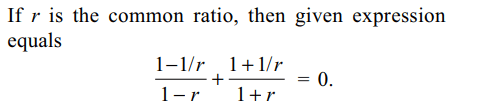5. If a, b, c are in G.P., then$\frac{1}{a^{2}-b^{2}}+\frac{1}{b^{2}}$     equals
a) $\frac{1}{c^{2}-b^{2}}$
b) $\frac{1}{b^{2}-c^{2}}$
c) $\frac{1}{c^{2}-a^{2}}$
d) $\frac{1}{b^{2}-a^{2}}$

Explanation: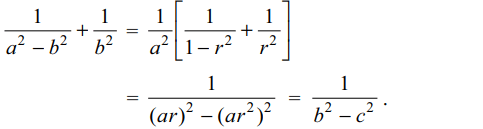6. If a, b, c, d are in H.P., then value of $\frac{a^{-2}-d^{-2}}{b^{-2}-c^{-2}}$     is
a) 1
b) 2
c) 3
d) 4

Explanation: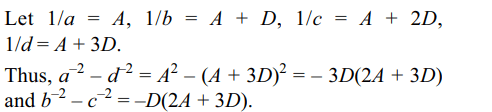7. Let a, b, c be distinct real numbers which are in G.P. If $x\epsilon R$  is such that a + x, b + x, c + x are in H.P., then x equals
a) a
b) b
c) c
d) (a + b + c)/3

Explanation: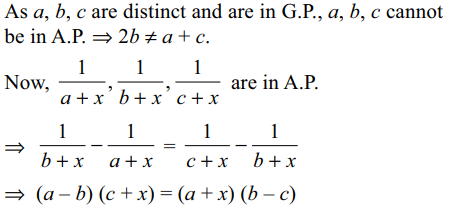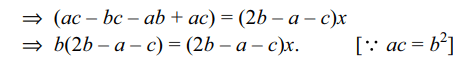8. Let a, b, c be in A.P., and
$\left(b – c\right) x^{2} + \left(c – a\right) x + \left(a – b\right) = 0$
and $2\left(a + c\right) x^{2} + \left(b+c\right) x = 0$
have a common root, then
a) $a^{2},b^{2},c^{2}$   are in G.P.
b) $a^{2},b^{2},c^{2}$   are in A.P
c) $a^{2},c^{2},b^{2}$   are in A.P
d) $a^{2},c^{2},b^{2}$   are in G.P.

Explanation: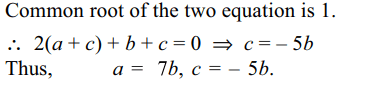9. Suppose a, b, c are in A.P. Let A, G be the arithmetic mean and geometric mean between a and b, A', G' be the arithmetic mean and geometric mean between b and c, then
a) $A^{2}+G^{2}=A'^{2}+G'^{2}$
b) $A^{2}-G'^{2}=A'^{2}-G^{2}$
c) $A^{2}-A'^{2}=G^{2}-G'^{2}$
d) $A^{2}+A'^{2}=G^{2}+G'^{2}$